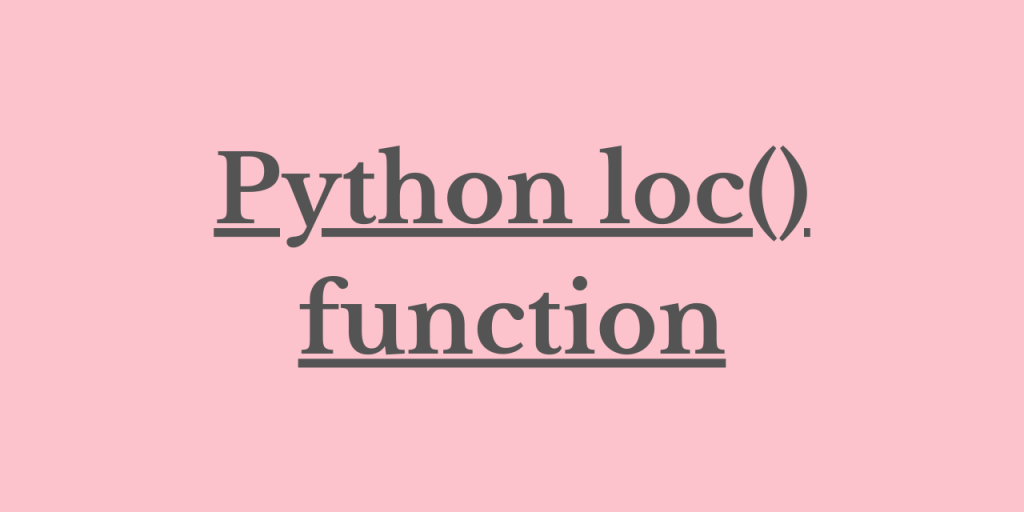# Python loc() function – Extract values from a datasetHey readers! In this article, we will be focusing on the functioning of Python loc() function in detail. So, let us get started!!

## Working of Python loc() function

Python comprises various modules that have in-built functions to deal with and manipulate the data values.

One such module is Pandas module.

Pandas module enables us to handle large data sets containing a considerably huge amount of data for processing altogether.

This is when `Python loc() function` comes into the picture. The loc() function helps us to retrieve data values from a dataset at an ease.

Using the loc() function, we can access the data values fitted in the particular row or column based on the index value passed to the function.

Syntax:

```pandas.DataFrame.loc[index label]
```

We need to provide the index values for which we want the entire data to be represented in the output.

The index label may be one of the below values:

• Single label – example: String
• List of string
• Slice objects with labels
• List of an array of labels, etc.

Thus, we can retrieve a particular record from a dataset based upon the index label using the loc() function.

Note: If the passed index is not present as a label, it returns KeyError.

Let us now focus on the implementation of the same using the below examples.

## Examples of Python loc() function

Let us first create a data frame with a set of data values using data frame in the Pandas module as shown below:

```import pandas as pd
data = pd.DataFrame([[1,1,1], [4,4,4], [7,7,7], [10,10,10]],
index=['Python', 'Java', 'C','Kotlin'],
columns=['RATE','EE','AA'])
print(data)
```

Dataframe:

```	RATE	EE	AA
Python	1	1	1
Java	4	4	4
C	7	7	7
Kotlin	10	10	10
```

Having created the data frame with a defined set of values, let us now try to retrieve a set of rows or columns having data values for a particular index as shown below:

### Extract One Row from a Data frame

```print(data.loc['Python'])
```

So, using the above command, we have extracted all the data values associated with the index label ‘Python’.

Output:

```RATE    1
EE      1
AA      1
Name: Python, dtype: int64
```

### Extract Multiple Rows from a Data frame

Let us now try to extract the data rows and columns associated with multiple indexes at the same time using the below command.

```print(data.loc[['Python','C']])
```

Output:

```          RATE  EE  AA
Python     1    1    1
C          7    7    7
```

### Extract Range of Rows using Python loc()

```print(data.loc['Python':'C'])
```

Here, we have used the slice object as with labels to display the rows and columns associated with the labels from ‘Python’ to ‘C’.

Output:

```          RATE  EE  AA
Python     1   1   1
Java       4   4   4
C          7   7   7
```

## Conclusion

By this, we have come to the end of this topic. Feel free to comment below, in case you come across any question.

For more such posts related to Python, Stay tune and till then Happy Learning!!